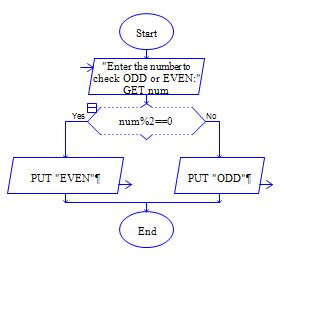# C Program to find whether the given number is odd or even number

Get a number num and check whether num is odd or even?

Sample Input 1:

45

Sample Output 1:

ODD

Sample Input 2:

56

Sample Output 2:

EVEN

#### Flow Chart DesignStrongly recommended to Solve it on your own, Don't directly go to the solution given below.

#include<stdio.h> int main() { //write your code here }

#### Program or Solution

``` #include<stdio.h> int main() { int num; printf("Enter two numbers:"); scanf("%d"&num); if(num%2==0) { printf("%d is even",num); } else { printf("%d is odd",num); } return 0; } ```

#### Program Explanation

Get input num from user using scanf statement

check whether the remainder of num divided by 2 is equal to 0 using if statement.

if it is 0, then print num is even using printf statement.

Else print num is odd using printf statement.